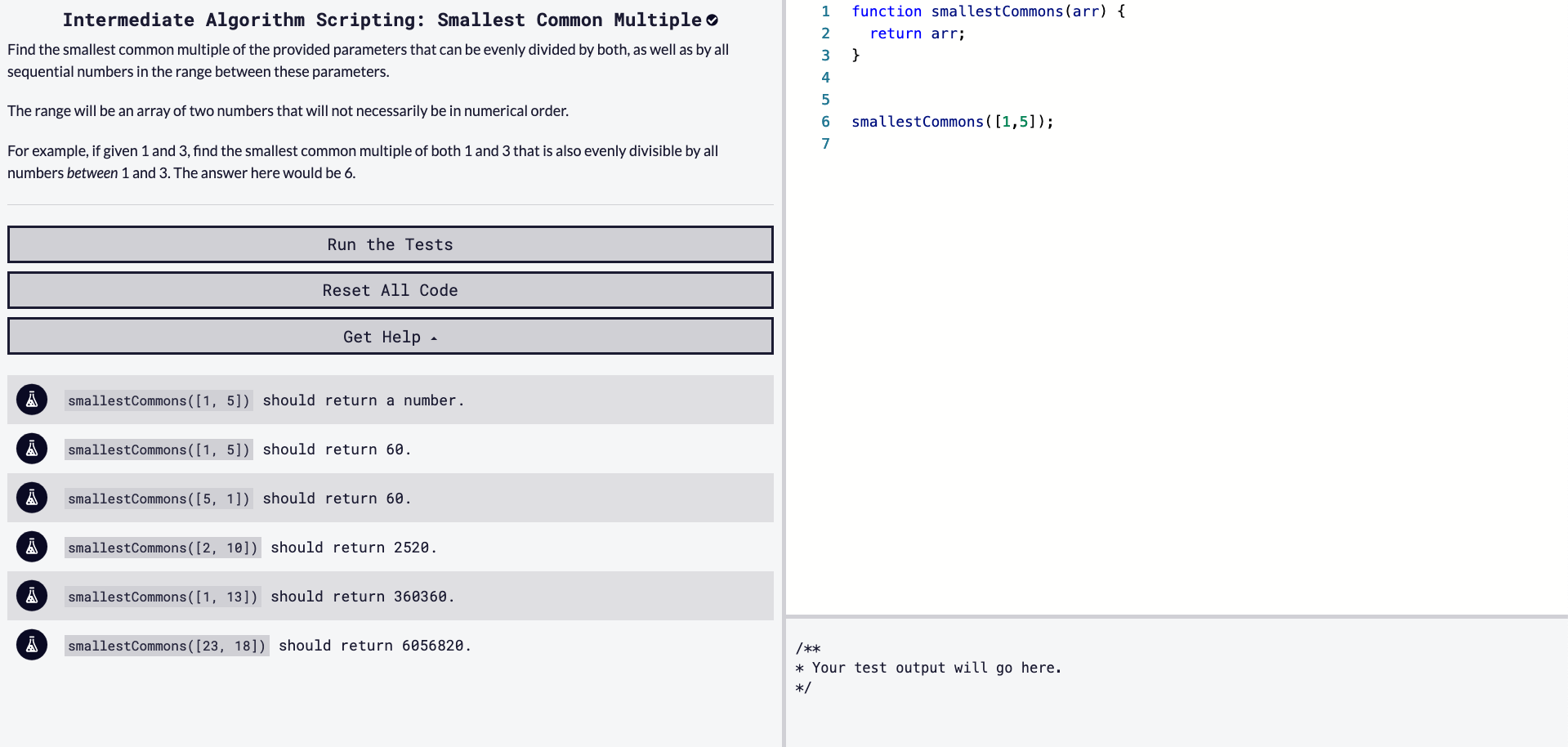# Solving "Smallest Common Multiple" / freeCodeCamp Algorithm Challenges

### 7/29/2020Let's solve freeCodeCamp's intermediate algorithm scripting challenge, 'Smallest Common Multiple'.

### Starter Code

``````function smallestCommons(arr) {
return arr;
}

smallestCommons([1,5]);
``````

### Instructions

Find the smallest common multiple of the provided parameters that can be evenly divided by both, as well as by all sequential numbers in the range between these parameters.

The range will be an array of two numbers that will not necessarily be in numerical order.

For example, if given 1 and 3, find the smallest common multiple of both 1 and 3 that is also evenly divisible by all numbers between 1 and 3. The answer here would be 6.

### Test Cases

• `smallestCommons([1, 5])` should return a number.
• `smallestCommons([1, 5])` should return 60.
• `smallestCommons([5, 1])` should return 60.
• `smallestCommons([2, 10])` should return 2520.
• `smallestCommons([1, 13])` should return 360360.
• `smallestCommons([23, 18])` should return 6056820.

# Our Approach

• We have one input, an array with two indexes, always positive numbers.
• We must return a number.

We have another math based challenge, just like yesterday's. We'll look more at the formula of what is commonly referred to as Least Common Multiple (LCM).

From ThinkMath, a multiple (of a number) is any product of that number and an integer.

It is often useful to know what multiples two numbers have in common. One way is to list (some of) the multiples of each and look for a pattern. For example, to find the common (positive) multiples of 4 and 6, we might list:

• Multiples of 4: 4, 8, 12, 16, 20, 24, 28, 32, 36, 40, 44, 48, ...
• Multiples of 6: 6, 12, 18, 24, 30, 36, 42, 48, 54, 60, ...

The numbers 12, 24, 36, and 48 appear on both of these lists (and more would appear if the lists were longer). They are common multiples. The least common multiple is the smallest of these: 12. All the other common multiples are, themselves, multiples of the least common multiple.

Source: ThinkMath

We'll touch on the math and the challenging part in more detail soon.

I'll start off the challenge by using `sort()` on `arr`, to make sure the bigger number is the first index.

``````arr = arr.sort((a,b) => b - a);
``````

sort() on MDN

Next, I'll save each value into their own variable. We can do that by destructuring `arr`.

``````let arr = [100, 50];
let [high, low] = arr;
console.log(high);
// 100
console.log(low);
// 50

let arr = [100, 50];
let high = arr;
let low = arr;
``````

Destructuring on MDN

So it looks like we will use a `for` loop to check on the divisibility of these two numbers (and the sequence of numbers in between). Before I start the loop, I will declare one more variable:

``````let multiple = high;
``````

I'm setting `multiple` to the larger variable, `high`.

For our `for` loop, it will run as long as `low` is smaller than `high`. See the below code -

``````for (let i = low; i < high; i++) {}
``````

So, looking at our test cases, let's disect `[5, 1]`. Without any code, what are the multiples (the answer should be 60):

5: 1, 5, 10, 15, 20, 25, 30, 35, 40, 45, 50, 55, 60, 65, 70

1: 1, 2, 3, 4, 5

So 5 is the smallest common multiple but we want a number that is evenly divisible by all the numbers between 1 and 5. This is the real challenge. So just doing some math without code, we can study the above numbers and determine 60 is the first number that is divisble evenly by 1, 2, 3, 4, 5.

For example, if given 1 and 3, find the smallest common multiple of both 1 and 3 that is also evenly divisible by all numbers between 1 and 3. The answer here would be 6.

So in our `for` loop, we can first check if `multiple` is not divisible by `low`. If it is not, we will add the value of `high` to `multiple`, then reduce our `i` variable and keep looping.

``````if (multiple % 1 !== 0) {
multiple += high;
i = low - 1;
}
``````

We can add an `else if` statement, if `i` equals `high`, we know we have found our answer. Let's see how this works step by step.

``````function smallestCommons(arr) {
arr = arr.sort((a,b) => b - a);
let [high, low] = arr;
let multiple = high;

for (let i = low; i < high; i++) {
if (multiple % i !== 0) {
multiple += high;
i = low - 1;
}
else if (i == high) {
return multiple;
}
return multiple
}
``````

Starting the `for` loop,

• `i = 1, multiple = 3`

• 3 / 1 = 0 reminder so we ignore the `if` statement (and also the `else if`)
• `i = 2, multiple = 3`

• 3 /2 = 1 remainder, so we add `high` to `multiple` and also set i = low - 1;
• i = 0 but next loop we i++
• `i = 1, multiple = 6`

• 6 / 1 remainder is 0 so we don't increment multiple
• `i = 2, multiple = 6`

• 6 / 2 remainder is 0 so we don't increment multiple
• next loop, with i++, i is no longer less than high so we exit the for loop

We return `multiple`

This may not be the most efficient solution!

# Our Solution

``````function smallestCommons(arr) {
arr = arr.sort((a,b) => b - a);
let [high, low] = arr;
let multiple = high;

for (let i = low; i < high; i++) {
if (multiple % i !== 0) {
multiple += high;
i = low - 1;
}
else if (i == high) {
return multiple;
}

return multiple;
}
}
``````